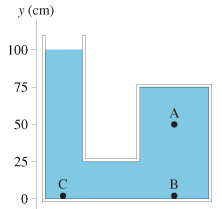# Problem: The container shown in the figure is filled with oil. It is open to the atmosphere on the left.(a) What is the pressure at point A?(b) What is the pressure difference between points A and B?(c) What is the pressure difference between points A and C?

###### FREE Expert Solution
99% (377 ratings)View Complete Written Solution
###### Problem Details

The container shown in the figure is filled with oil. It is open to the atmosphere on the left.(a) What is the pressure at point A?

(b) What is the pressure difference between points A and B?

(c) What is the pressure difference between points A and C?